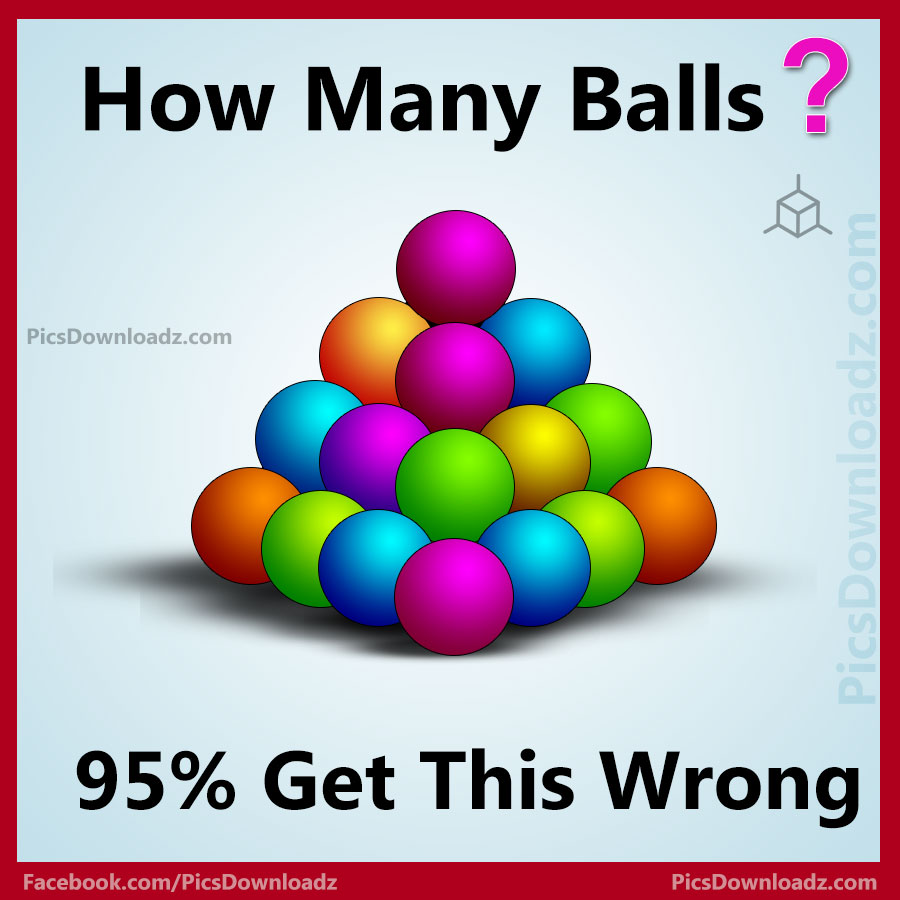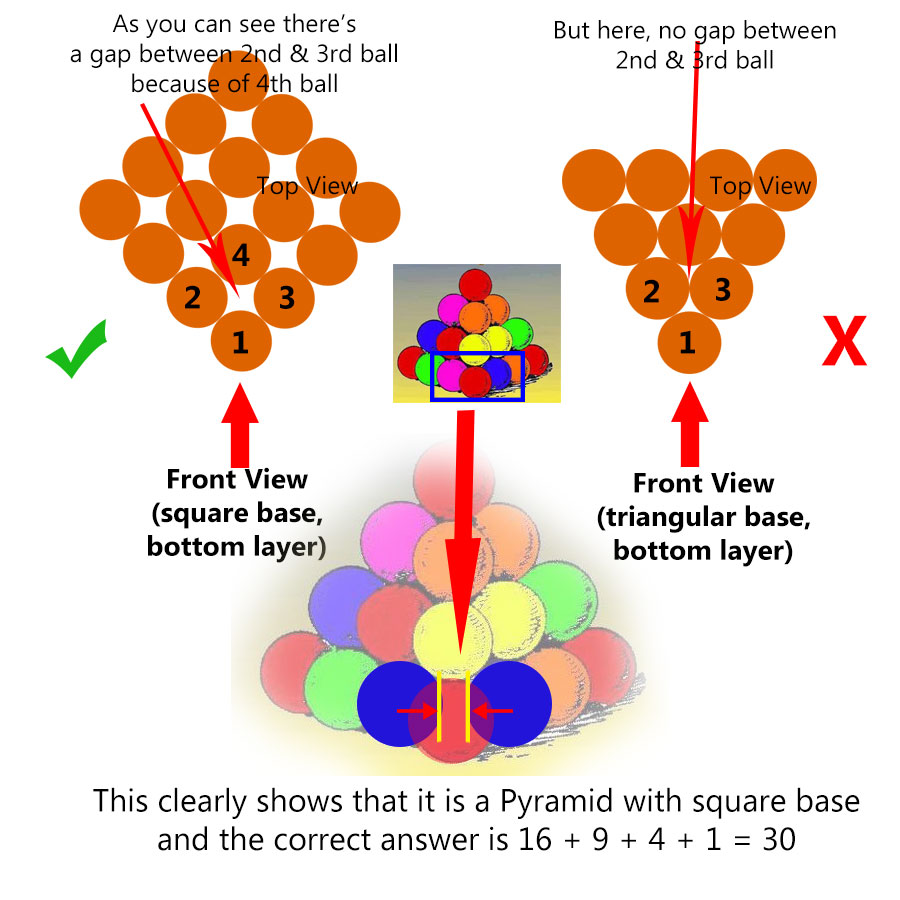This post may contain affiliate links. This means if you click on the link and purchase the item, We will receive an affiliate commission at no extra cost to you. See Our Affiliate Policy for more info.

# 95% get this wrong! How many Balls? Toughest Puzzle on the Internet!

19
34394

### Most Confusing riddle on internet: How many balls? Can you guess the correct number of balls in this puzzle image? Best Logical & viral math puzzle on the internet. 95% fail to guess the correct answer for this simple balls puzzle.

In this Puzzle, numbers of balls shown in the picture. You have to apply the logic and count, How many Balls in the Picture? Only Genius peoples can solve this puzzle. The Correct Answer & the Solution of this interesting Puzzle is given below! 😀

Let’s see how many of you can crack this genius logic puzzle.

## How Many Balls? Can You Solve it?Share this genius puzzle in your WhatsApp and facebook group let see how many of your friends can solve this logical puzzle. Like our FB page and join our puzzle group for more interesting brainteasers puzzle and question. Enjoy Take Care!

### Want to print or share on your blog?

Simply download this puzzle image and print it out. And for blog and website, you are free to use this image but you must attribute the image by linking to the original source, so we will be able to continue creating new puzzles & other amazing posts for you. <3

The image is in 3D shape, and as you can see the balls is in Pyramid Shape structure. The pyramid needs to be stable, so there are Hidden Balls in it.

Therefore Pyramid with a square base

⇒ 4*4 Base level = 16

⇒ First level = 9

⇒ Second level = 4

⇒ Top = 1

Total number of Balls = 16 + 9 + 4 + 1 = 30  Correct Answer

### Wrong Solution

For people, who consider these Balls Pyramid with the triangular base and get 20 as an answer are wrong. Because it’s clearly not a triangular base pyramid. why? check out the logic.Share & Comment! 😀

Search Items:-

Best Confusing Picture Puzzles, Tough Puzzles on internet, Viral Math Puzzles for kids, Best Brainteaser Puzzles images for adults, Fun Brainteaser Image, Tricky picture Puzzles, Interesting Math Puzzles, Only For Genius Math Puzzles, Difficult Question Puzzle, How many Balls in the Picture Puzzle, Counting Balls Puzzles, Number of balls Puzzles, Pyramid Balls Puzzles with Answer, Puzzles images with Solutions, Puzzles For WhatsApp, Puzzles For Facebook, Puzzles For Genius.

1.Larry

32 balls

2.Francis Lynah III

30 balls is right

3.Tom

HTom Lorence26

4.Dave

20 is also correct as it may not be a 4 sided pyramid but a 3 sided one. so the top ball is sat on 3 which sits on 6 which sits on 10. 1+3+6+10=20.

5.Tony

16 balls. It’s a drawing, what you see is what you get.

6.GILLIAN GOSS

I’m with Tony – 16. However, if we’re supposed to consider it in 3D, I’ve got 25.

7.Rhonda

16 on bottom
12 on the next row
4 on the next
1 on top
_______
33

8.Nannette Taylor

29…?

9.Fred

It’s 16 That you can see 25 total.

10.J.B.

30
16 on the bottom
9 on the next
4 on the next
1 on top

11.Raj Kashyap

12+8+5=25 is correct.

•Noor Jahan

25 balls

12.Dave

It’s based on the pharaoh puzzle, which is 20. Two columns of 4, and two groups of 6 balls.

13.Donald Parliament

Don I think there are 31

14.Mags

I think 25

15.Chris

It is an interesting puzzle…but the question is misleading -> How many balls?
There are many potential answers [are we to assume the structure is self supporting??]….How many balls…do I see? = 16. How many balls…. are needed (at a minimum?) to support this structure? Lets see: The top row is 1 ball. The next lower row can be either 3 balls or 4 balls as the top row appears to nest into the next lower row, but we are assuming the minimum so 3 balls. The next question is…how many balls would be required then to touch a row of 3 balls above…..the answer is 1 ball, so that row shows me 5 balls +1 hidden ball = 6 balls in that row. The next question then is how many balls would be needed on the bottom row to support the second row of 6 balls and all the rows above it…I see 7 balls plus how many additional balls (hidden would be required to carry the 3 rows above it?…I think the answer is 3 balls, so 7 shown +3 hidden = 10.
Total balls = 1+3+6+10 = 20 balls.

16.Mary

2 ball

17.Francis

1+4+8+12=25

18.Nabiha

25 balls